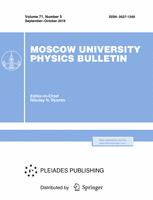Faculty of Physics
M.V.Lomonosov Moscow State University
Regular Article

Features of $^{19}$F–MRI research of Perftoranum$^{R}$ at 0.5, 7 and 11.7 T magnetic fields

D. V. Volkov$^1$, M.V. Gulyaev$^2$, O. S. Pavlova$^2$, N.V. Anisimov$^2$, Yu.A. Pirogov$^3$

Memoirs of the Faculty of Physics 2016. N 5.

• Article
Annotation

By exciting different parts of $^{19}$F–NMR–spectrum of Perftoranum$^{R}$ and experimentally the spectral range, which gives the maximum NMR–signal, was determined. It was shown that the preferable scanning method is pulse sequence RARE.

PACS:
82.56.Dj High resolution NMR
82.56.Hg Multinuclear NMR
82.56.Jn Pulse sequences in NMR
Rissian citation:
Д.В. Волков,  М.В. Гуляев, О.С. Павлова, Н. В. Анисимов, Ю.А. Пирогов.
Учен. зап. физ. фак-та Моск. ун-та. 2016. № 5. 165403.
Authors
D. V. Volkov$^1$, M.V. Gulyaev$^2$, O. S. Pavlova$^2$, N.V. Anisimov$^2$, Yu.A. Pirogov$^3$
$^1$Department of accelerator physics and radiation medicine, Faculty of Physics, Lomonosov Moscow State University Leninskie gory, 1, bldg. 2, Moscow 119991, Russia.
$^2$Laboratory of Magnetic Tomography and Spectroscopy, Faculty of Fundamental Medicine Lomonosov Moscow State University, Lomonosovskiy ave., 31, bldg. 4, Moscow 119192, Russia.
$^3$Department of Photonics and Microwave Physics, Faculty of Physics, Lomonosov Moscow State University Leninskie gory, 1, bldg. 2, Moscow 119991, Russia.Moscow University Physics BulletinScience News of the Faculty of Physics, Lomonosov Moscow State University

This new information publication, which is intended to convey to the staff, students and graduate students, faculty colleagues and partners of the main achievements of scientists and scientific information on the events in the life of university physicists.